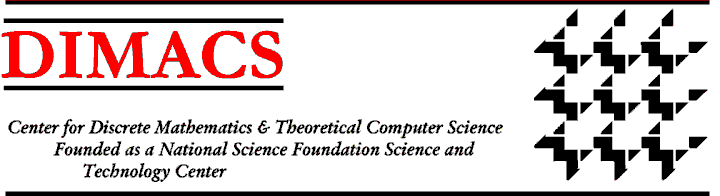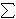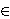### A Selection of Working Group Accomplishments: Working Group on Streaming Data Analysis and Mining I

A Windowed Data Stream Model for Detecting Patterns in Streaming Data.
In many areas of application, the sheer volume of data requires us to make a quick decision about it as it streams'' by. This is particularly true in applications of fraud detection in telecommunications, in surveillance in homeland security, and in financial transactions, as well as in astrophysics, environmental modeling, etc. We have been working on methods to detect patterns in streaming data. S. Muthukrishnan (Rutgers) and Mayur Datar (Stanford) developed a unique windowed data stream model'' that is based on observing a window'' of the last N observations. With storage space less than the window size N, they studied the problem of estimating the rarity'' of items in the window, a crucial problem in telephone credit card fraud detection and anomaly detection in counter-terrorism surveillance. They also studied the problem of estimating the similarity'' between two data stream windows, an important problem in sharing data between datasets obtained by different agencies/companies, a vital problem in the homeland security area. Dr. Muthukrishnan visited the NJ Office of Insurance Fraud in the Division of Criminal Justice to discuss analogous problems they are having. Muthukrishnan and Datar found novel, simple algorithms for estimating rarity and similarity in this windowed situation.

Constructing the First Known Data Stream Algorithm for Estimating Dominance of Multiple Signals.
Graham Cormode (University of Warwick) and S. Muthukrishnan (Rutgers) considered streams of multiple signals (i,ai,j) where the i's correspond to the domain, the j's index the different signals and i,ai,j gives the value of the j'th signal at point i. They addressed the problem of determining the dominance norms over the multiple signals, in particular the max-dominance norm defined asi maxj ai, j. Besides finding many applications, such as in estimating the worst case influence'' of multiple processes, for example in IP traffic analysis or electrical grid monitoring, this norm is a natural measure: it generalizes the notion of union of data streams and may be alternately thought of as estimating the L1 norm of the upper envelope of multiple signals. Cormode and Muthukrishnan constructed the first known data stream algorithm for estimating max-dominance of multiple signals. The algorithm is simple and implementable; its analysis relies on using properties of stable random distributions with small parameter alpha, which may be a technique of independent interest. They also showed that other dominance norms -- min-dominance sumiminjai,j, count-dominance (|i|ai>bi|) or relative-dominance (sumiai/max1,bi) -- are all impossible to estimate accurately with sublinear space.

Space Lower Bounds for Distance Approximation in the Data Stream Model. Michael Saks (Rutgers) and Xiaodong Sun (Rutgers) investigated the problem of approximating the distance between two d-dimensional vectors x and y in the classical data stream model. In this model, the 2d coordinates are presented as a stream'' of data in some arbitrary order, where each data item includes the index and value of some coordinate and a bit that identifies the vector ( x or y) to which it belongs. The goal is to minimize the amount of memory needed to approximate the distance. For the case of Lp-distance with p[1,2], there were known good approximation algorithms that run in polylogarithmic space in d (here one assumes that each coordinate is an integer with O(log d) bits). Saks and Sun proved that they do not exist for p>2. In particular, they proved a nearly optimal approximation-space tradeoff of approximating $L^\infty$ distance of two vectors. They showed that any randomized algorithm that approximates $L^\infty$ distance of two length d vectors within factor of $d^\delta$ requires $\Omega(d^{1-4\delta})$ space. As a consequence they showed that for $p>2/(1-4\delta)$, any randomized algorithm that approximate Lp distance of two length d vectors within a factor $d^{\delta}$ requires $\Omega(d^{1-\frac 2p-4\delta})$ space. The data stream model has been recognized recently as an important model, because it abstracts the problem of analyzing vast amounts of data streaming'' into a collection point. As for any model of computation, it is of interest to draw a dividing line between things that are efficiently computable in the model and things that are not. Previously other researchers had shown that approximating the distance of two vectors in this model could be done efficiently for standard Euclidean distance and L1 (Manhattan) distance. These algorithmic techniques did not help for the case of $L^\infty$ (max) distance. The work of Saks and Sun demonstrated that this latter problem is inherently unsolvable efficiently, provided a striking contrast with the other two cases. The lower bound follows from a lower bound on the two-party one-round communication complexity of this problem. This lower bound is proved using a combination of information theory and Fourier analysis.

M. Saks and X. Sun, Space Lower Bounds for Distance Approximation in the Data Stream Model, Proceedings 34th Annual ACM Symposium on Theory of Computing (STOC), 2002, 360-369.

A revised and expanded version has been submitted for journal publication.Index of Special Focus on Data Analysis and MiningDIMACS Homepage